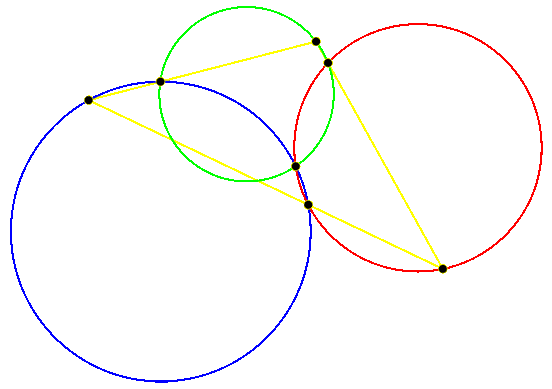# What is Geometer?Geometer is a free dynamic geometry program that runs on Macintosh or Windows and allows you to draw and modify euclidean geometry figures. Of course the author makes no guarantees about the program, but you will probably find it to be quite useful.

If you don't know what a dynamic geometry program is, click here for an animated demonstration of how Geometer can help visualize a theorem. (It's about 350K in size, so it will take a while over a telephone modem.)

If you'd just like to get an idea of the sorts of things Geometer can draw, look at the pictures in this Teacher's Tutorial. (Obviously the screen shots get more interesting toward the end of the tutorial.)

The features file gives an executive overview of what Geometer can do; the download page lets you download anything from the bare executable to the whole shooting match, and the sample diagrams can be loaded after you've got Geometer running on your system.

Here are some simple geometric constructions illustrated with Geometer diagrams. These would be suitable for an introductory high school course.
Geometric Constructions

Here's a step-by step illustration of how Geometer might be used to help discover and prove a theorem from Geometry:
Problem Solving with Geometer

Finally, if you want to try your hand at using Geometer to solve some moderately tough problems, check out the:
Geometer Problem Page